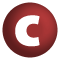# Random

#### class Random extends AnyRef

known subclasses: Random

1. AnyRef
2. Any

### Value Members

1. #### def equals(arg0: Any): Boolean

This method is used to compare the receiver object (`this`) with the argument object (`arg0`) for equivalence

This method is used to compare the receiver object (`this`) with the argument object (`arg0`) for equivalence.

The default implementations of this method is an equivalence relation:

• It is reflexive: for any instance `x` of type `Any`, `x.equals(x)` should return `true`.
• It is symmetric: for any instances `x` and `y` of type `Any`, `x.equals(y)` should return `true` if and only if `y.equals(x)` returns `true`.
• It is transitive: for any instances `x`, `y`, and `z` of type `AnyRef` if `x.equals(y)` returns `true` and `y.equals(z)` returns `true`, then `x.equals(z)` should return `true`.

If you override this method, you should verify that your implementation remains an equivalence relation. Additionally, when overriding this method it is often necessary to override `hashCode` to ensure that objects that are "equal" (`o1.equals(o2)` returns `true`) hash to the same `Int` (`o1.hashCode.equals(o2.hashCode)`).

arg0

the object to compare against this object for equality.

returns

`true` if the receiver object is equivalent to the argument; `false` otherwise.

definition classes: AnyRef ⇐ Any
2. #### def hashCode(): Int

Returns a hash code value for the object

Returns a hash code value for the object.

The default hashing algorithm is platform dependent.

Note that it is allowed for two objects to have identical hash codes (`o1.hashCode.equals(o2.hashCode)`) yet not be equal (`o1.equals(o2)` returns `false`). A degenerate implementation could always return `0`. However, it is required that if two objects are equal (`o1.equals(o2)` returns `true`) that they have identical hash codes (`o1.hashCode.equals(o2.hashCode)`). Therefore, when overriding this method, be sure to verify that the behavior is consistent with the `equals` method.

definition classes: AnyRef ⇐ Any
3. #### def nextASCIIString(length: Int): String

Returns a pseudorandomly generated String drawing upon only ASCII characters between 33 and 126

Returns a pseudorandomly generated String drawing upon only ASCII characters between 33 and 126.

4. #### def nextBoolean(): Boolean

Returns the next pseudorandom, uniformly distributed boolean value from this random number generator's sequence

Returns the next pseudorandom, uniformly distributed boolean value from this random number generator's sequence.

5. #### def nextBytes(bytes: Array[Byte]): Unit

Generates random bytes and places them into a user-supplied byte array

Generates random bytes and places them into a user-supplied byte array.

6. #### def nextDouble(): Double

Returns the next pseudorandom, uniformly distributed double value between 0

Returns the next pseudorandom, uniformly distributed double value between 0.0 and 1.0 from this random number generator's sequence.

7. #### def nextFloat(): Float

Returns the next pseudorandom, uniformly distributed float value between 0

Returns the next pseudorandom, uniformly distributed float value between 0.0 and 1.0 from this random number generator's sequence.

8. #### def nextGaussian(): Double

Returns the next pseudorandom, Gaussian ("normally") distributed double value with mean 0

Returns the next pseudorandom, Gaussian ("normally") distributed double value with mean 0.0 and standard deviation 1.0 from this random number generator's sequence.

9. #### def nextInt(n: Int): Int

Returns a pseudorandom, uniformly distributed int value between 0 (inclusive) and the specified value (exclusive), drawn from this random number generator's sequence

Returns a pseudorandom, uniformly distributed int value between 0 (inclusive) and the specified value (exclusive), drawn from this random number generator's sequence.

10. #### def nextInt(): Int

Returns the next pseudorandom, uniformly distributed int value from this random number generator's sequence

Returns the next pseudorandom, uniformly distributed int value from this random number generator's sequence.

11. #### def nextLong(): Long

Returns the next pseudorandom, uniformly distributed long value from this random number generator's sequence

Returns the next pseudorandom, uniformly distributed long value from this random number generator's sequence.

12. #### def nextString(length: Int): String

Returns a pseudorandomly generated String

Returns a pseudorandomly generated String. This routine does not take any measures to preserve the randomness of the distribution in the face of factors like unicode's variable-length encoding, so please don't use this for anything important. It's primarily intended for generating test data.

length

the desired length of the String

returns

the String

15. #### def toString(): String

Returns a string representation of the object

Returns a string representation of the object.

The default representation is platform dependent.

definition classes: AnyRef ⇐ Any

### Instance constructors

1. #### new Random()

Creates a new random number generator

Creates a new random number generator.

2. #### new Random(seed: Int)

Creates a new random number generator using a single integer seed

Creates a new random number generator using a single integer seed.

3. #### new Random(seed: Long)

Creates a new random number generator using a single long seed

Creates a new random number generator using a single long seed.